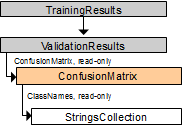# ConfusionMatrix Object (IConfusionMatrix Interface)

This object represents the confusion matrix for a classification model. The confusion matrix is a square table in which each cell contains the number of objects classified into category <column-name> while belonging to category <row-name>. The diagonal cells will contain the number of correctly assigned elements for each category.

The matrix size is the number of categories plus one, with the first column showing the number of objects in each category for which classification failed.

## Properties

Name Type Description
ClassNames StringsCollection, read-only The names of the categories in the order in which they appear. The rows correspond to the actual category, the columns — to the category predicted by the classification model.
Element Integer, read-only The matrix cell value.
ElementEx Integer, read-only The matrix cell value.
Size Integer, read-only The number of rows in the confusion matrix. It is equal to the number of categories plus 1: the extra column corresponds to the objects which the model was unable to classify.

## Related objects